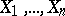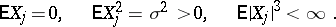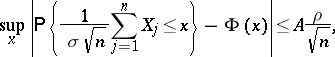# Berry-Esseen inequality

(diff) ← Older revision | Latest revision (diff) | Newer revision → (diff)

An inequality giving an estimate of the deviation of the distribution function of a sum of independent random variables from the normal distribution function. Letbe independent random variables with the same distribution such thatLetandthen, for any,whereis an absolute positive constant. This result was obtained by A.C. Berry  and, independently, by C.G. Esseen .

How to Cite This Entry:
Berry-Esseen inequality. Encyclopedia of Mathematics. URL: http://encyclopediaofmath.org/index.php?title=Berry-Esseen_inequality&oldid=16984
This article was adapted from an original article by V.V. Petrov (originator), which appeared in Encyclopedia of Mathematics - ISBN 1402006098. See original article